# Exam I Physics 101 Lecture 05 Free Fall

• Slides: 16
Download presentationExam I Physics 101: Lecture 05 Free Fall and Apparent Weight l Today’s lecture will cover Textbook Sections 4. 3, 4. 5 Check iclicker scores! James scholar paper topics due Fri Feb 19 Exam 1 is March 1 Office hours staffed 10 am – 8 pm on Mondays Physics 101: Lecture 5, Pg 1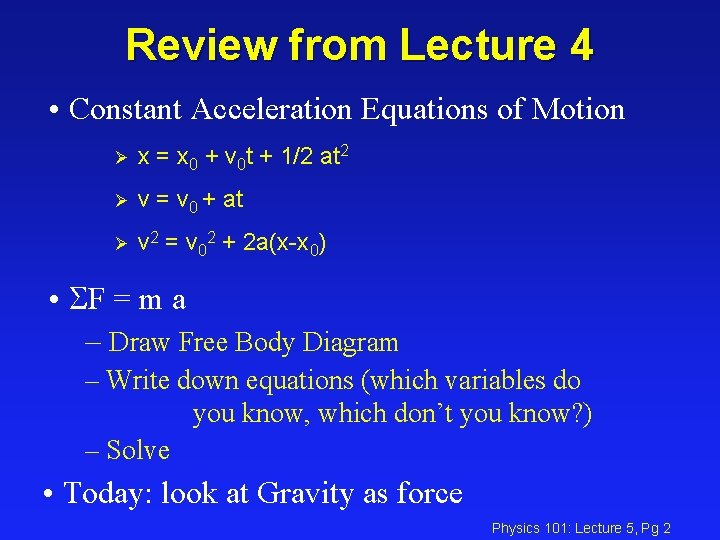Review from Lecture 4 • Constant Acceleration Equations of Motion Ø x = x 0 + v 0 t + 1/2 at 2 Ø v = v 0 + at Ø v 2 = v 02 + 2 a(x-x 0) • SF = m a – Draw Free Body Diagram – Write down equations (which variables do you know, which don’t you know? ) – Solve • Today: look at Gravity as force Physics 101: Lecture 5, Pg 2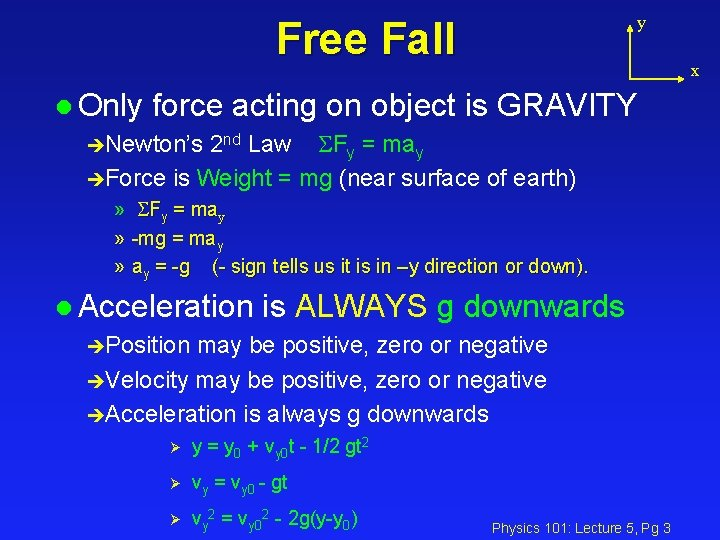y Free Fall x l Only force acting on object is GRAVITY èNewton’s 2 nd Law SFy = may èForce is Weight = mg (near surface of earth) » SFy = may » -mg = may » ay = -g (- sign tells us it is in –y direction or down). l Acceleration is ALWAYS g downwards èPosition may be positive, zero or negative èVelocity may be positive, zero or negative èAcceleration is always g downwards Ø y = y 0 + vy 0 t - 1/2 gt 2 Ø vy = vy 0 - gt Ø vy 2 = vy 02 - 2 g(y-y 0) Physics 101: Lecture 5, Pg 3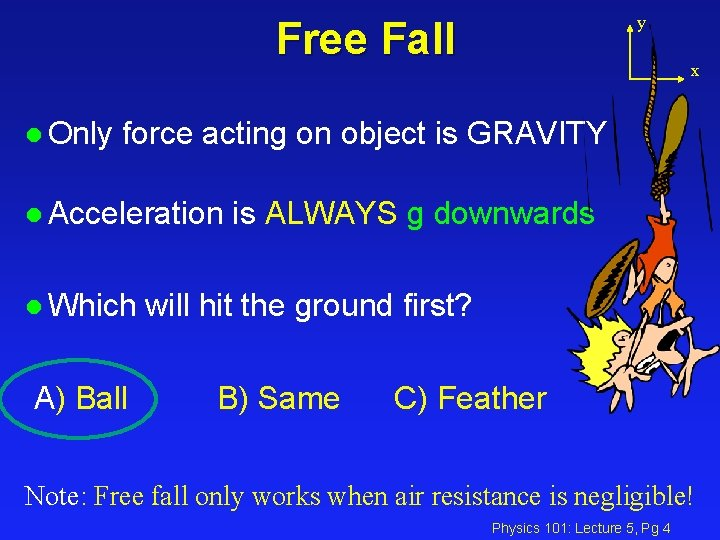y Free Fall l Only x force acting on object is GRAVITY l Acceleration l Which A) Ball is ALWAYS g downwards will hit the ground first? B) Same C) Feather Note: Free fall only works when air resistance is negligible! Physics 101: Lecture 5, Pg 4ACT l The speed of an object in free fall (Neglect Air Resistance) A. Always increases. is constant. Always decreases. May increase or decrease or be constant. May increase or decrease but is never constant. B. C. D. E. a = -g. Velocity becomes more and more negative. If v > 0, speed decreases. If v <= 0 speed increases. Physics 101: Lecture 5, Pg 5Free Fall ACTS Fred throws a ball 30 mph vertically upward. Which of the following statements are true about the ball’s velocity and acceleration. (Let up be the positive direction) On the way up? A) v < 0 B) v = 0 C) v > 0 A) a < 0 B) a = 0 C) a > 0 On the way down? Physics 101: Lecture 5, Pg 6ACT Fred throws a ball 30 mph vertically upward and then catches it again at the same height he threw it from. What is the speed of the ball when he catches it? (Neglect air resistance) 1) v < 30 mph 2) v = 30 mph Øvy 2 = vy 02 - 2 g(y-y 0) Øvy 2 = vy 02 3) v > 30 mph Physics 101: Lecture 5, Pg 7Free Fall Example Fred throws a ball 30 m/s vertically upward. What is the maximum height the ball reaches? How long does it take to reach this height? v 2 -vo 2 = 2 a y v = v 0 + a t y = (v 2 -vo 2 )/ (2 a) t = (v-v 0) / a = -302 / (2 * -9. 8) = (0 – 30 m/s )/ (-9. 8 m/s 2) = 46 m. = 3. 1 seconds Physics 101: Lecture 5, Pg 8ACT Dennis and Carmen are standing on the edge of a cliff. Dennis throws a basketball vertically upward, and at the same time Carmen throws a basketball vertically downward with the same initial speed. You are standing below the cliff observing this strange behavior. Whose ball hits the ground first? A. Dennis' ball B. Carmen's ball C. Same y = yo+vot + ½ a t 2 Dennis: 0 = H + vot - ½ g t 2 Carmen: 0 = H - vot - ½ g t 2 Carmen v 0 Dennis H v. A v. B Physics 101: Lecture 5, Pg 9ACT Dennis and Carmen are standing on the edge of a cliff. Dennis throws a basketball vertically upward, and at the same time Carmen throws a basketball vertically downward with the same initial speed. You are standing below the cliff observing this strange behavior. Whose ball is moving fastest when it hits the ground? A. Dennis' ball B. Carmen's ball Correct: C. Same v 2 = v 02 -2 g y Carmen v 0 Dennis H v. A v. B Physics 101: Lecture 5, Pg 10Apparent Weight y l Recall: SF = m a èConsider person accelerating up in an elevator. èDraw FBD N èApply NSL » N – mg = ma » N = m(g+a) mg • Apparent weight is normal force from scale or floor. • Note: in free fall a=-g so N=0 Physics 101: Lecture 5, Pg 11 x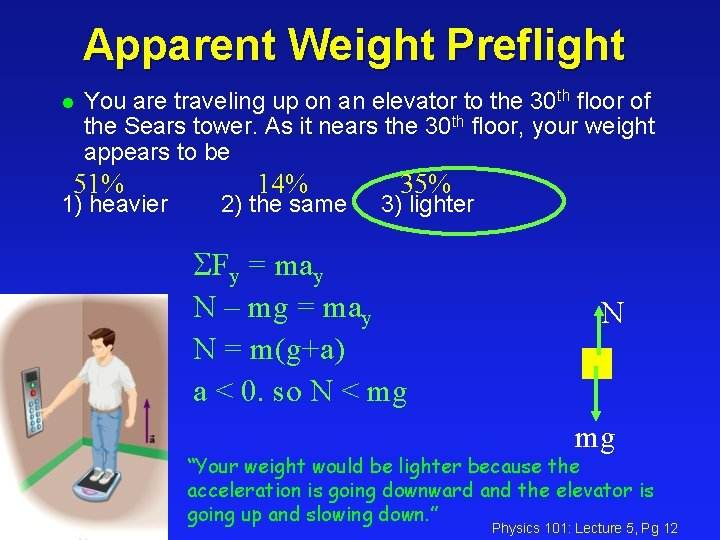Apparent Weight Preflight l You are traveling up on an elevator to the 30 th floor of the Sears tower. As it nears the 30 th floor, your weight appears to be 51% 1) heavier 14% 2) the same 35% 3) lighter SFy = may N – mg = may N = m(g+a) a < 0. so N < mg N mg “Your weight would be lighter because the acceleration is going downward and the elevator is going up and slowing down. ” Physics 101: Lecture 5, Pg 12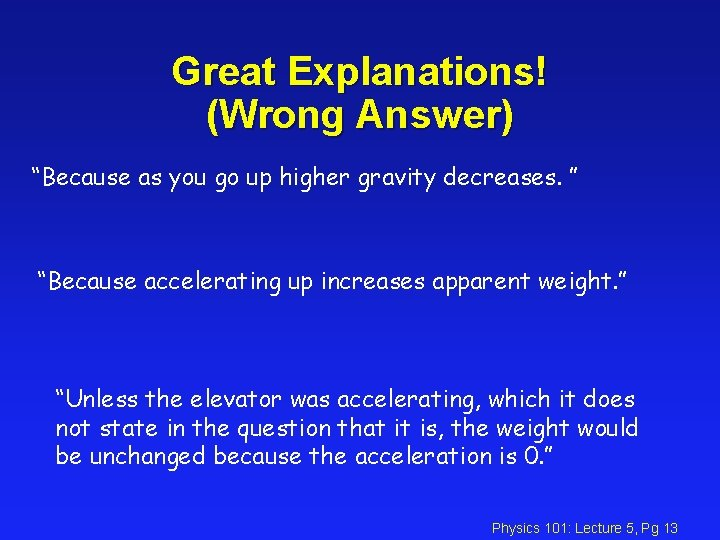Great Explanations! (Wrong Answer) “Because as you go up higher gravity decreases. ” “Because accelerating up increases apparent weight. ” “Unless the elevator was accelerating, which it does not state in the question that it is, the weight would be unchanged because the acceleration is 0. ” Physics 101: Lecture 5, Pg 13Apparent Weight Acts l A person has mass 50 kg. What is their apparent weight when they are riding on N = m(g+a) an elevator 1. Going up with constant speed 9. 8 m/s a = 0 so N= mg = 490 Newtons 2. Going down with constant speed 9. 8 m/s a = 0 so N= mg = 490 Newtons 3. Accelerating up at a rate of 9. 8 m/s 2 a = +9. 8 m/s so N= 2 mg = 980 Newtons 4. Accelerating down at a rate of 9. 8 m/s 2 a = -9. 8 m/s so N= 0 mg = 0 Newtons Physics 101: Lecture 5, Pg 14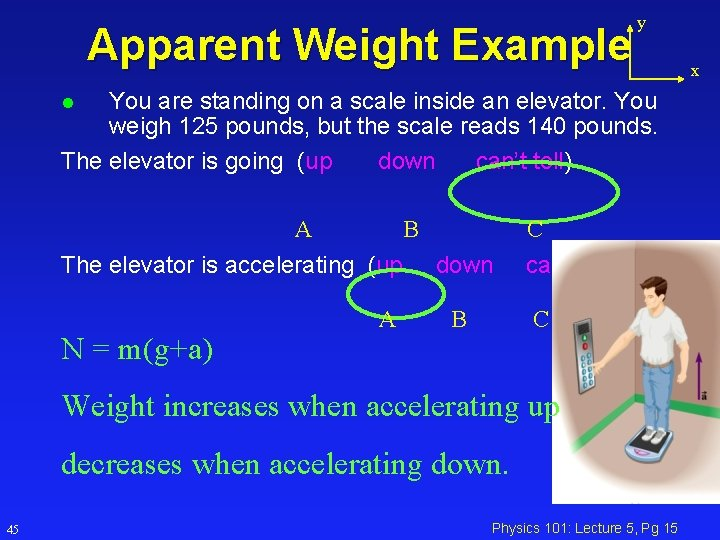Apparent Weight Example y You are standing on a scale inside an elevator. You weigh 125 pounds, but the scale reads 140 pounds. The elevator is going (up down can’t tell) l A B The elevator is accelerating (up down N = m(g+a) A B C can’t tell) C Weight increases when accelerating up decreases when accelerating down. 45 Physics 101: Lecture 5, Pg 15 x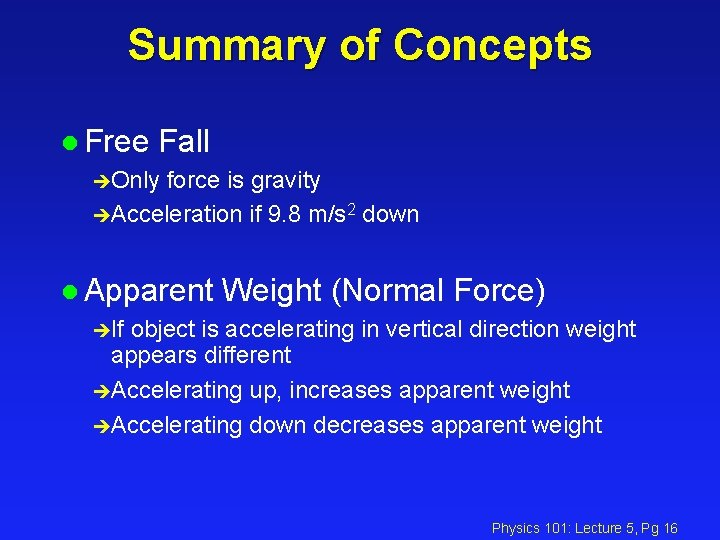Summary of Concepts l Free Fall èOnly force is gravity èAcceleration if 9. 8 m/s 2 down l Apparent Weight (Normal Force) èIf object is accelerating in vertical direction weight appears different èAccelerating up, increases apparent weight èAccelerating down decreases apparent weight Physics 101: Lecture 5, Pg 16